Deutsche Version● Adding acoustic levels of sound sources

Addition of SPLSum of levelsvoltage , sound, and noise

Summing up to ten incoherent or uncorrelated  noise sources

 Incoherent or noncoherent means the signals of the overdubbed channels are irrelative like a violin and a trumpet, that means having no correlative relationship. Sometimes we say uncorrelated when we mean incoherent. Given two incoherent sources their combined effect is the sum of their acoustic power.

Combining decibels - adding up to 10 incoherent acoustic levels ●

The decibel calculator can be used to combine the levels of up to ten incoherent (noncoherent)
electric or acoustic sources when the level of each source is known in decibels (dB).

 Level 1 dB Level 2 dB Level 3 dB Level 4 dB Level 5 dB Level 6 dB Level 7 dB Level 8 dB Level 9 dB Level 10 dB Total Level dB
 Fill in as many sound level boxes as necessary (max 10) and then click the calculate bar, to get the calculated sum. Provided, that each sound source has its own random phasing.

Adding Amplitudes and Levels (coherent and incoherent)The ten octave bands of our hearing range

The formula for the sum level of sound pressures of n incoherent radiating sources is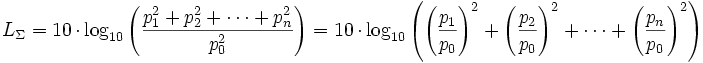The reference sound pressure p0 is 20 µPa = 0.00002 Pa = 2 × 10−5 Pa (RMS) ≡ 0 dB.

From the formula of the sound pressure level we find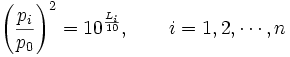This inserted in the formula for the sound pressure level to calculate the sum level shows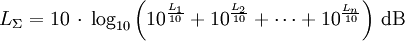LΣ = Total level and L1, L2, ... Ln = sound pressure level of the separate sources in dBSPL.
Incoherent means: lacking cohesion, connection, or harmony. It is not coherent.

For example, adding of threedecibel values, that means levels 94.0 + 96.0 + 98.0: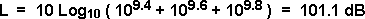Table for combining decibel levels

Difference between the two levels to be added in dB
 0 1 2 3 4 5 6 7 8 9 10 3.01 2.54 2.12 1.76 1.46 1.19 0.97 0.79 0.64 0.51 0.41
Difference between the two levels to be added in dB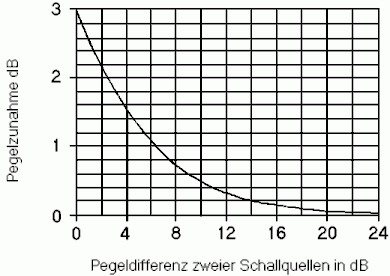Level difference between the two sound sources

 source 1 dB source 2 dB source 3 dB source 4 dB total dBAdding of equal loud incoherent sound sources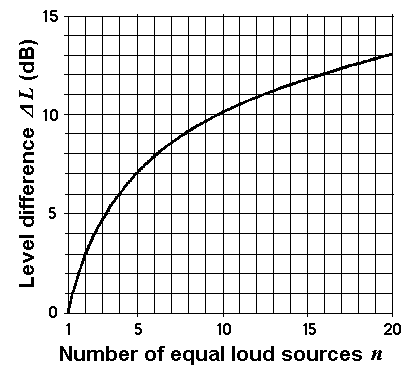Level increase Δ L for n equal loud sound sources Number of n equal loud sound sources Level increase Δ L in dB 1 0 2 3.0 3 4.8 4 6.0 5 7.0 6 7.8 7 8.5 8 9.0 9 9.5 10 10.0 12 10.8 16 12.0 20 13.0

Formulas: Δ L = 10 × log n  or  n = 10(ΔL/10)
Δ L = level difference; n = number of equal loud sound sources.

 n = 2 equally loud incoherent sound sources result in a higher level of 10 × log10 2 = +3.01 dB compared to the case that only one source is available. n = 3 equally loud incoherent sound sources result in a higher level of 10 × log10 3 = +4.77 dB compared to the case that only one source is available. n = 4 equally loud incoherent sound sources result in a higher level of 10 × log10 4 = +6.02 dB compared to the case that only one source is available. n =10 equally loud incoherent sound sources result in a higher level of 10 × log10 10 = +10.00 dB compared to the case that only one source is available.

Adding (combining) levels of equal loud sound sources

 To use the calculator, simply enter a value. The calculator works in both directions of the ↔ sign.

 Equal loud incoherent sound sources Number of sound sources n ↔ Increase of level Δ L   dB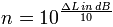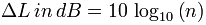The total level in dB is the level of one sound source plus the increase of level in dB.

 Example: The measurable noise of a motorcycle is at a certain distance 60 dB (A). How big is the total level of 4 motorcycles with the same volume? Solution: 60 dB (A) + 10 log 4 = 60 + 6 = 66 dB (A). If you are doing noise measurements of motorcycles you should at least consider the "honesty" of the dBA-readings without low frequencies.

 You can easily add up coherent and incoherent sound level and sound pressure values. It is often desired to add the psychoacoustic perceived loudness or volume. See:

 How many decibels (dB) level change is double, half, or four times as loud? How many dB to appear twice as loud (two times)? Here are all the different ratios. Ratio means "how many times" or "how much" ... Doubling of loudness.

 Level change Volume Loudness Voltage Sound pressure Acoustic Power Sound Intensity +40 dB 16 100 10000 +30 dB 8 31.6 1000 +20 dB 4 10 100 +10 dB 2.0 = double 3.16 = √10 10 +6 dB 1.52 times 2.0 = double 4.0 +3 dB 1.23 times 1.414 times = √2 2.0 = double - - - - ±0 dB - - - - - - - - 1.0 - - - - - - - - - - - 1.0 - - - - - - - - - - - 1.0 - - - - - −3 dB 0.816 times 0.707 times 0.5 = half −6 dB 0.660 times 0.5 = half 0.25 −10 dB 0.5 = half 0.316 0.1 −20 dB 0.25 0.100 0.01 −30 dB 0.125 0.0316 0.001 −40 dB 0.0625 0.0100 0.0001 Log. size Psycho size Field size Energy size dB change Loudness multipl. Amplitude multiplier Power multiplier

 Ratio Change in Sound Loudness Level Change in Sound Pressure Level Change in Sound Power Level 20 +43.22 dB +26.02 dB +13.01 dB 15 +39.07 dB +23.52 dB +11.76 dB 10 +33.22 dB +20 dB +10 dB 5 +23.22 dB +13.98 dB +6.99 dB 4 +20 dB +12.04 dB +6.02 dB 3 +15.58 dB +9.54 dB +4.77 dB 2 +10 dB +6.02 dB +3.01 dB - - - - - 1 - - - - - - - - - ±0 dB - - -- - - - - - ±0 dB - - - -- - - - ±0 dB - - -- - 1/2 = 0.5 −10 dB −6.02 dB −3.01 dB 1/3 = 0.3333 −15.58 dB −9.54 dB −4.77 dB 1/4 = 0.25 −20 dB −12.04 dB −6.02 dB 1/5 = 0.2 −23.22 dB −13.98 dB −6.99 dB 1/10 = 0.1 −33.22 dB −20 dB −10 dB 1/15 = 0.0667 −39.07 dB −23.52 dB −11.76 dB 1/20 = 0.05 −43.22 dB −26.02 dB −13.01 dB

Noise

 Noise is annoying, harassing and unwanted sound. It is not a physical phenomenon, but only mental processes change a sound to noise. There are a number of definitions of noise. Important ones are: 1 - the acoustic ratio that characterize the noise and by measurable physical sizes, such as the amplitude or the sound pressure level, frequency, and the time behavior of the sound, can be described. 2 - the situational ratio, i.e. location, time and situation in which the person is situate during the occurrence of the noise, and the relation to the activities, intentions and the current being of the person who is exposed to the noise. 3 - the personal ratio of the person who is exposed to the noise, with their acquired cognitive and emotional implications for the sound source. The fact that noise is not only dependant on physically measurable sizes, but "of more", makes the derivation of methods and calculation methods for the objective description to a problem and explains the problems of noise control, which are often found between the measured noise values and the perceived harassment. Kurt Tucholsky wrote aptly: "Our own dog does not make noise, it only barks.

 Castrated sound level values in weighted dBA are added the same way like sound level values in unweighted dB.

 Pro audio equipment often lists an A-weighted noise spec – not because it correlates well with our hearing – but because it can "hide" nasty hum components that make for bad noise specs.   Words to bright minds: Always wonder what a manufacturer  is hiding when they use A-weighting. *)